Pascal Implementation by Steven Pemberton and Martin Daniels

# Chapter 4: Code Generation

## Routines: Alignquot, align, genlabel, mes, putic, gen0, gen1, gen2, gentypindicator, gen0t, gen1t, gen2t, load, store, loadaddress, genfjp, genujpxjp, gencupent, checkbnds, putlabel

Code generation can be split into several parts:

• Output of individual instructions: mes, putic, gen0, gen1, gen2, gentypindicator, gen0t, gen1t, gen2t, genfjp, genujp4p, gencupent
• Generating labels: genlabel, putlabel
• Assigning addresses to variables: alignquot, align
• Generating checking code: checkbnds

Code is produced in symbolic form. Each line output is a comment or a label definition, an instruction or an end assembly line.

A comment is a line beginning with the letter I followed by a number: this is purely for the human reader, and just numbers every tenth instruction output.

For example

``I 10``

(Routine putic does this, and is its only task.)

Label definitions are lines beginning with the letter `L` followed by a number, optionally followed by an equals sign followed by another number. For example:

``L 10``
``L 12=4``

The first case is a code label, and is the destination of a jump or call within code. The second case defines a value for use by `ENT` instructions (`ENT` instructions are generated before the value is known).

Instruction lines start with a space followed by a three-letter name optionally followed by other information. An instruction can be 'typed' or 'untyped', and can have zero, one or two parameters.

An example of an untyped instruction without parameters is the stop instruction:

``STP``

Typed instructions are qualified by an extra letter giving information on the type of its operands. For example

`EQUI` compares two integers for equality

`EQUR` compares two reals.

Parameters consist of a P and a Q parameter, either of which may be absent. Typically Q is the major parameter, for instance the address of a variable, with P qualifying it in some way, such as which region to find it in. Examples:

`LAO 9` Load onto the stack the address of the variable at address 9 in the outer region.

`LDA 0 5` Load the address of the variable at address 5 in the local region.

`LDOI 10` Load the contents of the outer integer variable at address 10.

`LODI 1 5` Load the contents of the integer variable at address 5 of the region one level out.

An end assembly line consists solely of the letter Q:

``Q``

## Output of Individual Instructions

The names of these routines have a loosely adhered to coding for their names: the word gen, followed by a digit, optionally followed by a t. The digit indicates the number of parameters, and the t means 'typed'.

## Routine gen0, lines [1833-7]

This is the simplest of this set of routines, and a good place to start. It is used to output untyped instructions with no parameters:

`ABI`, `ABR`, `SQI`, `SQR`, `TRC`, `ODD`, `CHR`, `EOF`, `FLT`, `NOT`, `SGS`, `UNI`, `MPI`, `FLO`, `MPR`, `INT`, `DVR`, `DVI`, `MOD`, `AND`, `NGI`, `NGR`, `ADI`, `ADR`, `SBI`, `SBR`, `DIF`, `IOR`, `INN`, `UJC`, `STP`.

 Fop is the index of the instruction to be output, and is used to index the array mn , to give its name, and cdx , to give its effect on the stack (see later).

 Prcode is the boolean indicating whether code is to be output or not. Puttic simply outputs a comment line in the code every tenth instruction.

Mn is initialised at [3890-905] with the names of the instructions, so this writeln prints the name of the required instruction.

 Variable ic, the instruction counter, is incremented for each instruction output. Mes measures the effect that this instruction has on the runtime stack, in terms of operands put on or taken off the stack.

### Notes

1. Note that the ':4' in the writeln on  is not strictly necessary.
2. All the procedures for outputting instructions contain code similar to this. If this code, except using a write instead of a writeln, were gathered into a procedure called, say, gen, then all the other procedures could use it. In particular, gen0 would then look like
```procedure gen0(fop: oprange);
begin
gen(fop);
writeln(prr)
end;
```

## Routine mes, lines [1825-8]

At run-time the main program, and each invocation of a routine, has associated with it a 'stack frame' which contains the data areas for the routine.These areas are:

1. Result space for the result, should the routine be a function;
2. Certain system values needed for the upkeep of the frame;
3. Space for any parameters;
4. Space for any local variables;
5. A local stack space for holding partial results when evaluating expressions.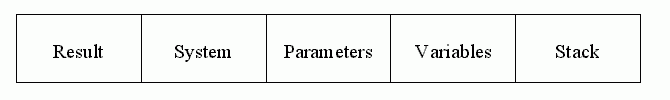The maximum size necessary for each of these areas can be calculated at compile-time, and mes is used for calculating the size of the local stack area.

Each instruction may have an effect on this local stack at run-time. For instance `LDC`, 'load constant', loads a constant on to the top of the stack, and so can be said to have an effect of +1. Similarly, `ADI` takes the top two stack items and replaces them by their integer sum, so this can be said to have an effect of -1.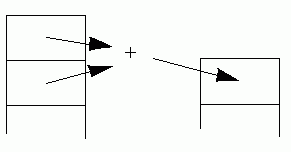Similarly all other instructions can be so classified, with zero for instructions with no effect.

This information is recorded in the array cdx initialised [3948-63].

In the same way, the standard functions and procedures have an effect on the stack, and these effects are recorded in the array pdx, initialised [3964-9]. The P-code names of the standard routines are held in the array sna, initialised [3880-5].

When a routine is compiled, there are two variables, topnew and topmax, initialised to lcaftermarkstack . Topnew indicates the current size of the local stack area, adjusted for every instruction output, topmax the largest value topnew has had in the current routine and therefore the required size of the local stack within the frame.

 Calculate the new value of topnew.

 Update topmax if necessary.

### Notes

1. The type of i should be oprange.
2. Topnew and topmax are initialised to lcaftermarkstack as this is the amount needed on the stack to call a procedure or function (= result space + system space), and the compiler assumes that every routine will call another. However, there is no need for this assumption if the cdx value for the `MST` (mark stack) instruction, generated for every routine call, is changed from zero to lcaftermarkstack.
3. Since mes does not have any type information (for example, for an `LDC` it does not know what type of constant is being loaded) it assumes the worst, and multiplies the change value by the size of the largest possible item that can be loaded on the stack, maxstack. The value of maxstack is the maximum of intsize, realsize, charsize, boolsize, ptrsize, and setsize, rounded to a multiple of stackal if necessary (see [34-56]).
4. The fact that the compiler assumes that every routine will call another, and that every change in the stack is multiplied by the largest simple type, and therefore more often than not vastly overestimates the size of the local stack, may seem to waste a lot of space. However, careful study of the procedure call mechanism in the interpreter will show that this space is seldom wasted, since new stack frames start at SP and not EP.
5. Values are never left on the stack between statements. Therefore topnew is always back to its initial value at the end of a statement.

## Routine gen1, lines [1839-66]

This routine is used for untyped instructions with one parameter (Q): `CSP`, `LCA`, `LAO`, `IXA`, `MST`, `MOV` and for `RET` which is typed with no parameters.

[1844-8] For the instruction `CSP` (call standard procedure), the effect of this instruction depends on which routine is being called, so pdx is used instead of cdx. Fp2 is the index of the standard routine being called.

[1850-9] Instruction `LCA` loads the address of a string. Here fp2 indexes an array of strings, and the characters of the string are output, surrounded by single quotes. Note that the string is padded out with spaces to make it exactly strglgth characters long.

 Instruction `RET` is actually a typed instruction, with a single character: P for procedures, and I, R, C, B, A for integer, real, character, boolean, and pointer functions respectively. Fp2 is just the ord of the character. However, gen1 is only called for the `RETP` case. Gen0t is used for the others.

 In all other cases, fp2 is just a value to be printed, for example

`MOV 6`

### Notes

1. This routine is used for too many diverse purposes. The cases for `CSP`, `LCA`, and `RET` should each have their own routine; it is pure contortion fitting them here.
2. The `LCA` case needs greater study, because of its use of the array cstptr.

Referring to the declaration of cstptr , there is a comment indicating that it is used for non-integer constants (real, string, and set; chars are treated as integers anyway), so that they can be treated as integers within the compiler. (There is also a confusing mention of a nonexistent procedure writeout; more on that later).

However, locating all the places where cstptr is used, the following cases emerge:  in gen1,  and  in gen2, where the constant stored in cstptr is printed out; and , ,  where a value is added to the array (printing an error message if full). But in these three latter cases, putting a value in is immediately followed by a call to either gen1 or gen2 using that value. Cstptrix the index into cstptr is never decremented, and cstptr is never used except in these six cases.

In other words, all the values stored are used once and once only, immediately after they are stored, and so cstptr need not be an array, but only a single variable - 64 elements are completely wasted, and error 254 need never occur!

In fact, this array is probably a left-over from a previous version of the compiler. Throughout the compiler there are clues - like the comment at , and the restriction that goto's may not lead out of a routine - that once upon a time the compiler stored the code of a routine in an array, and printed it out at the end of the routine. This probably included filling in the addresses of goto's as well, and when this organisation was changed, the storing of constants was overlooked.

Anyway, the cure for all this is to scrap cstptr completely, delete [1849-59], and introduce a new procedure genlca(fcp: csp); and replacing [2015-20] by genlca(cval.valp) and similarly for gen2 and the calls around  and .

3.  the ':1 'is not needed since sval[k] is of length 1 anyway.

## Routine gen2, lines [1868-1902]

This routine should be used for untyped instructions with two parameters (P and Q); however the only one that conforms to this is `LDA`. The others are:

`EQU`, `GEQ`, `GRT`, `LEQ`, `LES`, `NEQ`

which are typed with no parameters unless the type is M when there is one, and

`LDC`

which is typed with one parameter, except for `LDCN` which has none.

[1874-5] For `LDA` (load address, fop = 50), output the two parameters. Gen2 is never called with fop = 45, 54,or 56 (`CHK`, `LOD`, `STR`).

[1876-80] The compare instructions `EQU`, `GEQ`, `GRT`, `LEQ`, `LES`, and `NEQ`. The type character is printed, and if this is M, for string comparisons, the length of the string is also printed.

[1881-98] LDC (load constant). In this case fp1 indicates what type of constant is to be loaded (1 integer, 2 real, 3 boolean, 4 nil (in which case fp2 is not used), 5 set, and 6 character), and fp2 is the constant to be loaded, or a pointer to it.

### Notes

1. Again this routine is heavily overloaded; it should be split into separate routines for `LDA`, the comparison instructions, and for `LDC`.
2. You might consider whether for consistency `LCA`, which loads the address of a string (the value of a string is never loaded), should really be a `LDC` typed for string, and let the interpreter deal with the difference, especially since the interpreter already does special things for reals, sets and large integers.
3.  The ':3'is unnecessary.
4.  The ':3' is a mistake. If sethigh is greater than 99 (which is quite possible) then k may well be a three digit number, in which case subsequent values would run into each other. A better coding would be

`if k in pval then write(' ', k:1)`
5. Fp2 on  should be printed with width 11.

## Routine gentypindicator, lines [1904-23]

This routine is used from gen0t, gen1t, gen2t to output the type character of typed instructions.

 The type character for integer is 'i'.

 For boolean 'b'.

 For character 'c'.

 Enumerations apart from boolean are treated as integer.

 'r' for real.

 For subranges, gentypindicator is called recursively for the type of the subrange.

 's' for sets.

 'm' (multiple) for arrays and records.

 It should never be possible for this routine to be called for files, tagfields, and variants, so this would be a compiler error.

### Note

[1909-16] could be written more simply as

```if fsp=boolptr then write(prr, 'b')
else if fsp=charptr then write(prr, 'c')
else if fsp=realptr then write(prr, 'r')
else write(prr, 'i') (*intptr and enumerations*)```

since it does not repeat the 'i' case (see [3060-5]).

## Routines gen0t, gen1t, gen2t, lines [1925-56]

These are all used for typed instructions. Gen0t for instructions with no parameters:

`STO`, `ORD`, and `RET`,

gen1t for instructions with one parameter:

`LDO`, `IND`, `SRO`, `INC`, and `DEC`,

and gen2t for instructions with two parameters:

`LOD`, `STR`, `CHK`.

### Notes

1.  This prints fp1 in width 3 if it is less than 100, otherwise in width 8. Only `CHK` will have a first parameter larger than maxlevel .
2. Instruction `CHK` is used to check that the value on top of the stack is within the limits of the two parameters, for example

`CHKI 0 9`

would check that the integer is between 0 and 9. However `CHKA`, for checking addresses, does not need two parameters, since the correct range of values is determined by the interpreter not the compiler, except for whether the address is allowed to be nil or not. `CHKA` is generated from two places,  when dereferencing pointers, where the first parameter of 1 indicates that the address being checked may not be nil, and  when assigning pointers, where the zero indicates nil is acceptable. In fact these two calls are the only major uses of maxaddr, which could easily be eliminated.

## Routines genfjp, genujpxjp, gencupent, lines [2036-59]

These are for the instructions

`FJP`, `UJP`, `XJP`, `CUP`, and `ENT`.

Despite its name, genujpxjp is also used in one place for `FJP` . Otherwise the routine names reflect their uses.

These five instructions all share the property that they have a label parameter, indicated in the code by the letter `L` followed by an integer. For example,

`FJP L 10`

### Notes

1. Lines [2037-9] should not be here as their purpose is semantic analysis. They should be put in a procedure of their own, for example, checkbool, or expanded in-line at the three places genfjp is called. If this is done than genfjp and genujpxjp can be combined into one routine, say genjump, which has the added advantage of removing the slightly dirty use of genujpxjp for the `FJP`.
2. You might prefer error 135 rather than 144 at .
3. In fact the letter `L` before the label number is redundant, and can only be for human readability, since it is always known from context whether a parameter is a label or not (for instance the parameter of `FJP` is always a label, so ```FJP 10``` would be sufficient).

If this letter were removed, then these three routines could be removed, and gen1 and gen2 used instead. Of course, this would also imply a slight change in the interpreter.

## Routines genlabel, putlabel, lines [847-50, 2076-8]

These two routines are for creating labels to be referred to in the code, and outputting them.

 Intlabel, declared , initialised to zero , is just incremented each time genlabel is called, thus delivering a unique integer for each label.

 A generated label is output. (This is done only once for each label).

### Notes

1. Genlabel, being part of code generation, is clearly in the wrong place in the compiler.
2. Genlabel could be a function, replacing code like [1722-3]

`genlabel(lbname); pfname:=lbname`

with

`pfname:=genlabel`
3. All other procedures beginning "gen" output something to the code file. Perhaps createlabel would be a better name.

When assigning an address to a variable, two values have to be taken into account:

1. the amount of space needed by a variable of that type, and
2. the 'alignment constant' for that type.

Both these values of course depend on the target machine, which is why the compiler is parameterised with a set of these values for all the simple types [34 et seq.].

For example, some machines require four store units to hold an integer, and require that it be stored starting on an even store boundary. This would be reflected by values of 4 for intsize and 2 for intal.

When addresses are assigned to objects, they are assigned as offsets relative to a fixed point. So the first item is assigned the initial offset, and subsequent offsets increase from there.

As an example, suppose that integers need 2 units on an even boundary, and characters need one unit on any boundary. Then intsize and intal are 2, charsize and charal are 1. Also, assume the initial offset is zero. Now, space is to be allocated for two integers, a character, and another integer.

The first integer can be assigned offset zero, and the displacement incremented by intsize.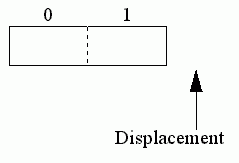Now the next integer can start at offset 2, as this is an even boundary: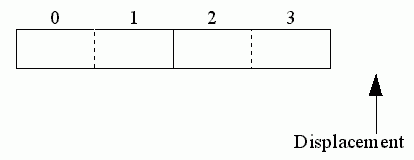The next item is a character, which may start at any boundary: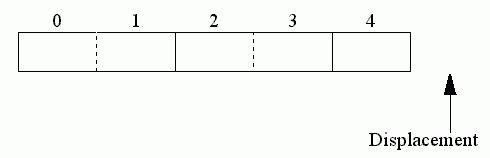Now the next item is an integer, and the current displacement is odd, so it has to be aligned to the next even boundary before allocating the address, so that location 5 is wasted: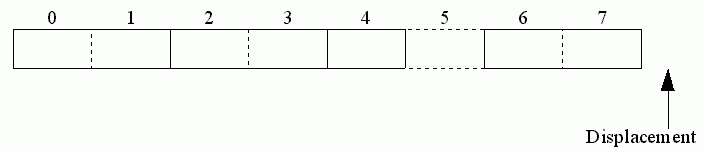All objects are allocated in this way: variables, parameters, fields of records, and elements of arrays.

For variables, there is a global variable lc that holds the current displacement within the current routine.

There are two routines to achieve address assignment: alignquot [646-66] for calculating the alignment value for an object, and align [668-74] for making sure the current displacement is a multiple of the alignment.

For instance, to allocate an integer variable in the current procedure would need

```align(intptr, lc);
lc:=lc+intsize```

All procedures and functions use the first few locations of their data space for system values and function results, so that the initial value of the displacement for these cases is not zero, but the value called lcaftermarkstack.

## Routine alignquot, lines [646-66]

 Default of 1.

[652-6] Standard types: get the value from the appropriate constant.

 Parameters to procedures. Because of the way they are evaluated by putting them on the stack, they have an alignment constant independent of their types.

 The alignment of a subrange is that of its rangetype.

[659-61] Pointers, sets, files.

 The alignment of an array is that of its element type.

 Records have an alignment constant independent of their fields.

 Alignquot is not called for variants and tag fields.

### Notes

1. The parmptr case is a bit of a misuse of the routine which is otherwise used for types.
2. The first 3 lines could be rephrased as

```if fsp=nil then alignquot:=i
else with fsp^ do```
3. The default is 1, since the alignment value is used as the divisor of mod in align , and therefore must not be zero.
4. The value for records is a worst-case simplification. If a record only consists of, say, character fields then it only needs to be aligned to a character boundary, and in general only needs to be the smallest common multiple of the alignment values for its fields. However rather than work this value out, the compiler uses the value recal , which is the smallest common multiple of all possible alignment values.
5. This routine is called every time an object is aligned. An alternative to this would be to calculate the value once and for all and store it with the type along with its size.

## Routine align, lines [668-74]

The workings of this routine are not immediately apparent. It is required to make sure that flc, the current displacement, is a multiple of k the required alignment value. The effect wanted is

```while (flc mod k) <> 0
do flc:=flc+1```

but without using a loop.

Since flc may already be a multiple of k, the problem can be restated as finding the next multiple of k that is greater than (flc - 1).

The next multiple of k below or equal to a value X is X - X mod k

Therefore the multiple of k above X is

X - X mod k+k.

Substituting (flc - 1) for X gives

(flc-1) - (flc-1) mod k + k.

However, flc at its smallest may be zero, so (flc-1)can be negative, and Pascal's mod is not defined for negative operands. So since

(a mod b) = ((a+b) mod b),

(flc - 1) - (flc - 1 + k) mod k + k.

Rearranging

(flc-1+k) - (flc-1+k) mod k.

This has been simplified to

``` l:=flc-1;
flc:=l+k - (l+k) mod k```

### Note

A better simplification would be

```l:=f-1+k;
f:=l-l mod k```

This procedure makes sure that code has been generated to completely load the current expression onto the stack.

 If typtr is nil, a compilation error occurred with this expression, and so no code need be generated for it.

[1964-81] Generate code to load a constant: boolean , character , integer or enumeration , nil , real , and set . (String constants are loaded in loadaddress.)

[1982-8] Code to load a variable.

 Load a variable at the outermost level, that is, a global variable.

 Load a variable local to some routine. Level - vlevel is the number of levels out from this level. Level is the current level, vlevel the level that the variable was declared at. Therefore a difference of zero means the variable is local to this routine, a difference of one means it comes from the surrounding routine, and so on.

 Code to load a value indirectly, via an address on the top of the stack.

 Set the kind to expression.

### Notes

1. The instruction `LDO`, that loads a global variable, is an optimisation, since `LOD` could still be used here. The same holds for the other two global accessing instructions `LAO` and `SRO`.

## Routine store, lines [1995-2006]

Unlike load and loadaddress which both use the global attributes gattr, store is passed the attributes it is to use via the formal parameter fattr. This is because for instance with the statement

`i:=1`

by the time that store is called, gattr refers to the right-hand side, whereas store needs the attributes of the left-hand side, which must therefore be saved and passed to the routine (see , , , ).

 Fattr.kind is always varbl.

 If typtr is nil then some compilation error occurred.

 Store into a direct global variable.

 Store into a direct non-global variable.

 Loadaddress is called for indirect variables prior to calling store, and sets idplmt to zero . Therefore if idplmt is not zero it is a compiler error.

 Store indirect.

This section then, is for loading the address of a string constant, for which there is a special instruction `LCA` (load constant address).

[2025-6] If access = indrct then an address is already loaded. More code only needs to be generated for the case of a field of this variable being accessed (for example a[i].left), in which case the address only needs to be incremented by the offset of the field, using an INCA instruction (increment address).

Nilptr is a structure with form pointer, used in gentypindicator  to generate the A of `INCA`.

 Reset the attributes of gattr.

### Note

As mentioned before, there is no need for a separate `LCA` instruction. `LDCM` would do as well, and the assembler could differentiate as necessary.

## Routine checkbnds, lines [2062-73]

This generates code to check if a value is in a required range. It is only ever called if debug is true, i.e. if the doption has been set. It is called from two places:  to check an actual parameter fits a formal parameter, and  to check the right hand side of an assignment fits the left, if the left is a subrange variable.

Fsp  is the type of the target variable (for example a in a:=b).

### Notes

1. Other productions of the instruction `CHK` occur at

 for array subscripts

 for dereferencing a pointer

 for assigning a pointer

 for the controlling expression of a case statement.

2. Unfortunately, the generation of checking code is far from optimal. For instance a call to a procedure like

`p('+');`

or an assignment

`ch:='+';`

would both generate code to check that '+' was a character. You might like to consider what changes would be necessary to improve on this. See (Welsh, 1978) for an interesting article on optimal checking code.

Pascal Implementation by Steven Pemberton and Martin Daniels